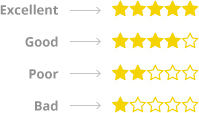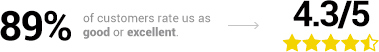On moving to Feefo 3.0, your current Feefo four-point score will automatically convert to a five-star rating.

The simplest way to look at the conversion process is to look at an example. If we assume you have the following number of Feefo reviews under the four-point system:

• 10 excellent reviews
• 7 good reviews
• 2 poor reviews

Under the four-point system, Feefo's merchant score was calculated as the percentage of customers who rate you as good or excellent. Using this example, 17 out of the 19 reviews are good or excellent resulting in a Feefo rating of 89%.

Under the five-star system, the rating is calculated as follows:

Firstly, the previous four ratings will map to stars:To calculate the new average rating, the following expression will be used:

• Each excellent review = 5
• Each good review = 4
• Each average review = 3
• Each poor review = 2
• Each bad review = 1

Using the above example, this produces the following calculation:In conclusion, for this example, the conversion results in: